# Linear Regression in Machine Learning: Hands-on Project Guide

Linear Regression in Machine Learning is a statistical analysis used to predict the relationship between two variables. It is a supervised machine learning algorithm with a continuous predicted output and constant slope.

You can use Linear Regression to predict values within a continuous range rather than classifying them.

In simpler words, Imagine you have a scatter plot of data points, and you want to draw a straight line that best captures the underlying trend in the data. That’s what linear regression is.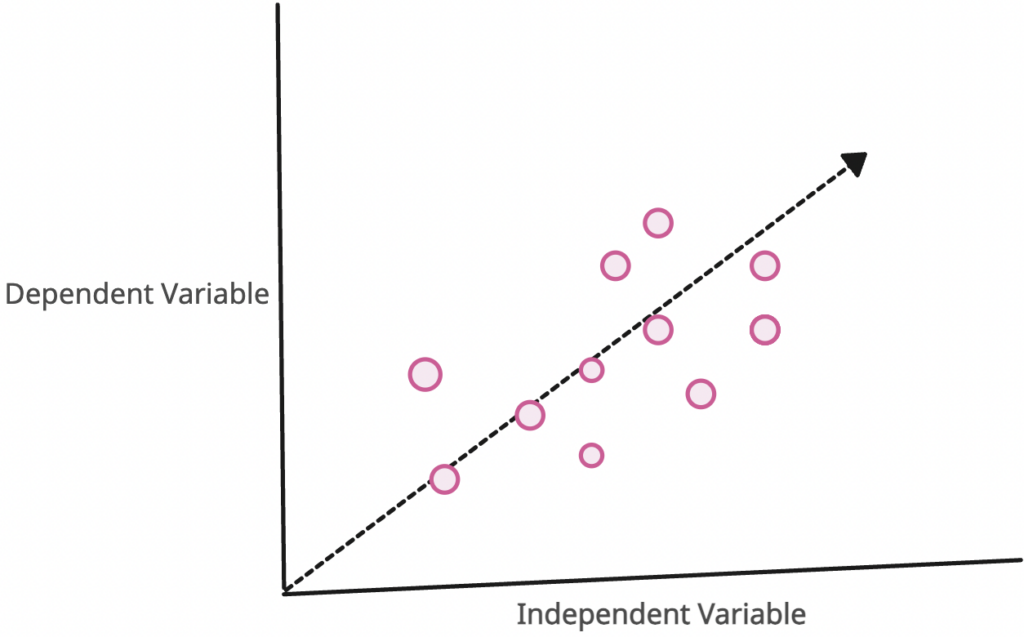The graph above presents the linear relationship between the output(y) and predictor(X) variables.

The dotted (—>) line is the best-fit straight line. Based on the given data points, we plot a line that best fits the points.

### What is the best-fit line?

The best-fit line is a line that fits the given scatter plot in the best way.

## Evaluation Metrics for Linear Regression

To evaluate the linear regression model, there are two main metrics.

1. Coefficient of Determination or R-squared (R2)
2. Root Mean Squared Error (RSME) and Residual Standard Error (RSE)

The strength of any linear regression model can be assessed using various evaluation metrics.

These evaluation metrics usually measure how well the model generates the observed outputs.

## Real-time Project implementation of the Linear Regression Model

Before starting any project, setting up a virtual environment is important.

This keeps the project’s dependencies separate from your global Python environment, ensuring reproducibility and avoiding potential conflicts.

### Step 1: Setting up a Virtual Environment

``````pip install virtualenv

virtualenv linear_regression_env

source linear_regression_env/bin/activate
``````

### Step 2: Install all the necessary libraries

``````pip install numpy pandas matplotlib seaborn scikit-learn
``````

### Step 3: Data Gathering

To implement a Linear regression model, we will need a Dataset, and Kaggle has the world’s best datasets that you can use to train your machine learning model.

For this tutorial, we will use Kaggle’s Boston Housing Dataset. You can check it out here.

### Step 4: Load the dataset

To import and convert a DataSet to DataFrame, you can use the Pandas.read_csv() method.

``````import pandas as pd

# Display the first few rows
``````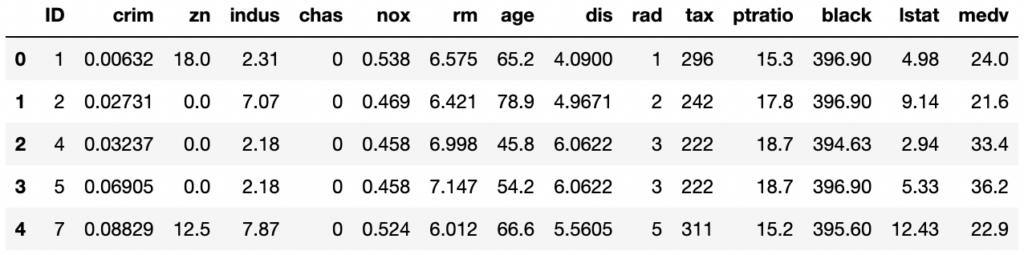### Step 5: Data Cleaning and Pre-processing

To check for missing values in the dataset, we will use the DataFrame.isnull() method and then apply the .sum() method to count the missing values row-wise.

``````# Check for missing values
missing_values = data.isnull().sum()

missing_values
``````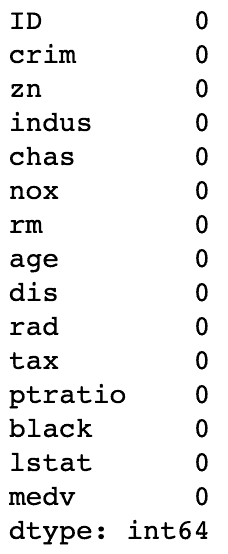That means the dataset is clean and has no missing values.

### Step 6: Exploratory Data Analysis (EDA)

EDA is important in understanding the data and its underlying patterns, distributions, and relationships. Let’s start with some basic visualizations.

1. Distribution of the Target Variable (medv): Visualize the distribution of the median value of homes using a histogram.
2. Correlation Matrix: It will help us understand the relationships between different features and the target variable.
3. Scatter Plots: We will plot scatter plots of some features against the target variable to check the linearity visually.

#### 6.1: Visualization of Distribution of the Target Variable (medv)

``````import matplotlib.pyplot as plt
import seaborn as sns

# Set the style for seaborn
sns.set_style("whitegrid")

# Plot the distribution of the target variable 'medv'
plt.figure(figsize=(10, 6))
sns.histplot(data['medv'], bins=30, kde=True)
plt.title('Distribution of Median Home Value (medv)')
plt.xlabel('Median Home Value (\$1000s)')
plt.ylabel('Frequency')
plt.show()
``````

Output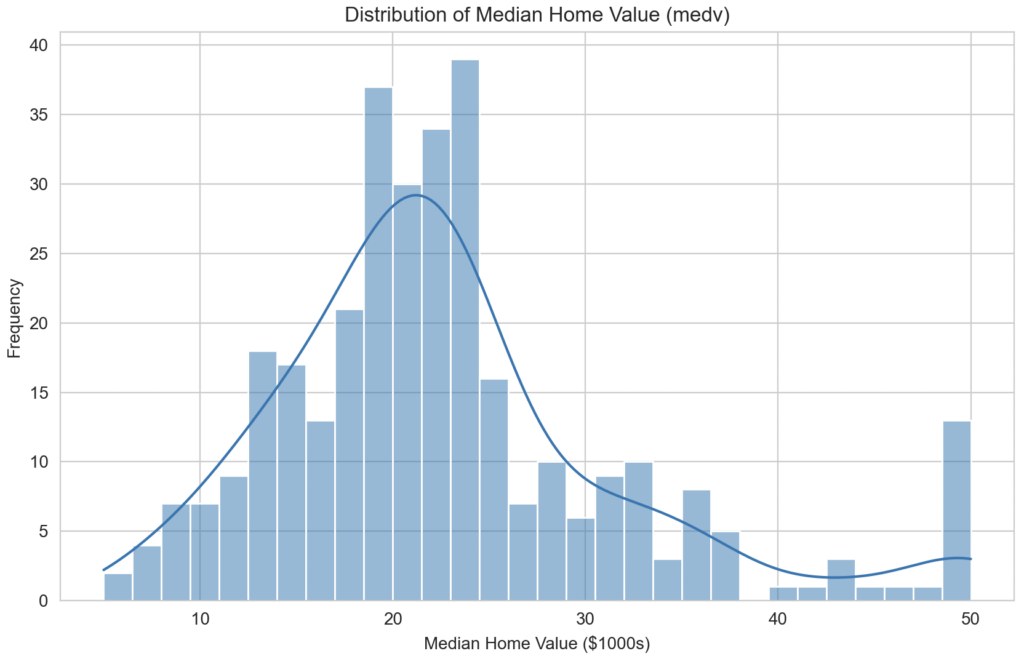The histogram provides insights into the distribution of the median home values. Most houses have a median value of around \$20,000 to \$25,000 (remember, the values are in the \$1000s).

#### 6.2: Visualize the correlation matrix

``````# Plot the correlation matrix
correlation_matrix = data.corr()
plt.figure(figsize=(14, 10))
sns.heatmap(correlation_matrix, annot=True, cmap="coolwarm", linewidths=.5)
plt.title('Correlation Matrix')
plt.show()``````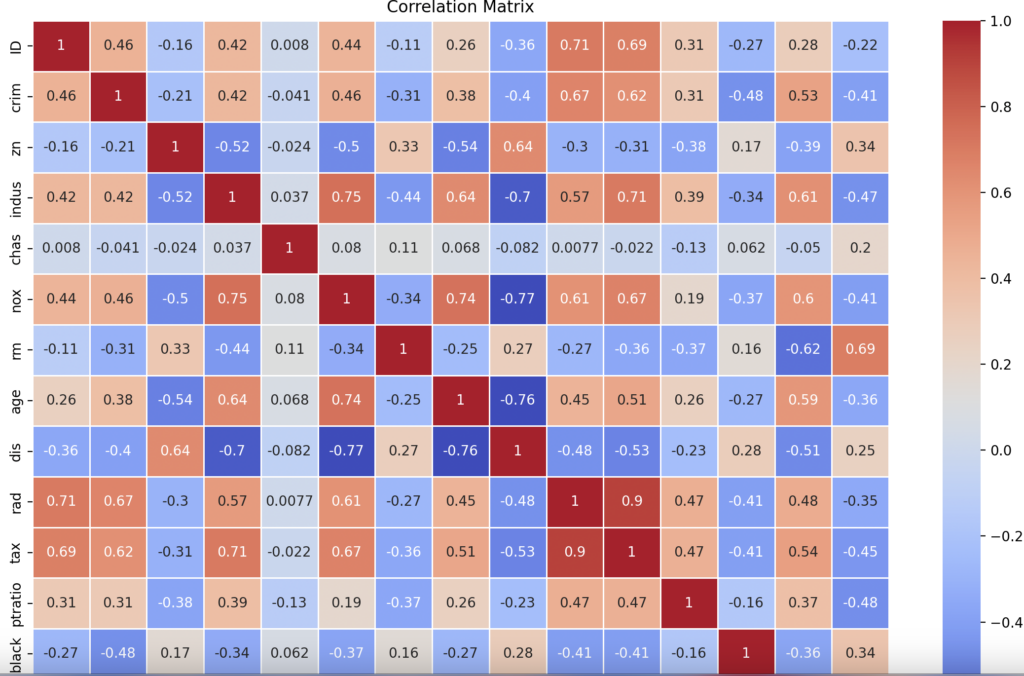#### 6.3: Visualize the scatter plot

Using scatter plots, let’s visualize the relationship between medv and some strongly correlated features.

``````# Scatter plot of rm vs medv
plt.figure(figsize=(12, 6))
sns.scatterplot(x=data['rm'], y=data['medv'], alpha=0.6, edgecolor=None)
plt.title('Number of Rooms vs Median Home Value')
plt.xlabel('Average Number of Rooms')
plt.ylabel('Median Home Value (\$1000s)')
plt.show()

# Scatter plot of lstat vs medv
plt.figure(figsize=(12, 6))
sns.scatterplot(x=data['lstat'], y=data['medv'], alpha=0.6, edgecolor=None, color='red')
plt.title('Percentage of Lower Status Population vs Median Home Value')
plt.xlabel('Percentage of Lower Status Population')
plt.ylabel('Median Home Value (\$1000s)')
plt.show()
``````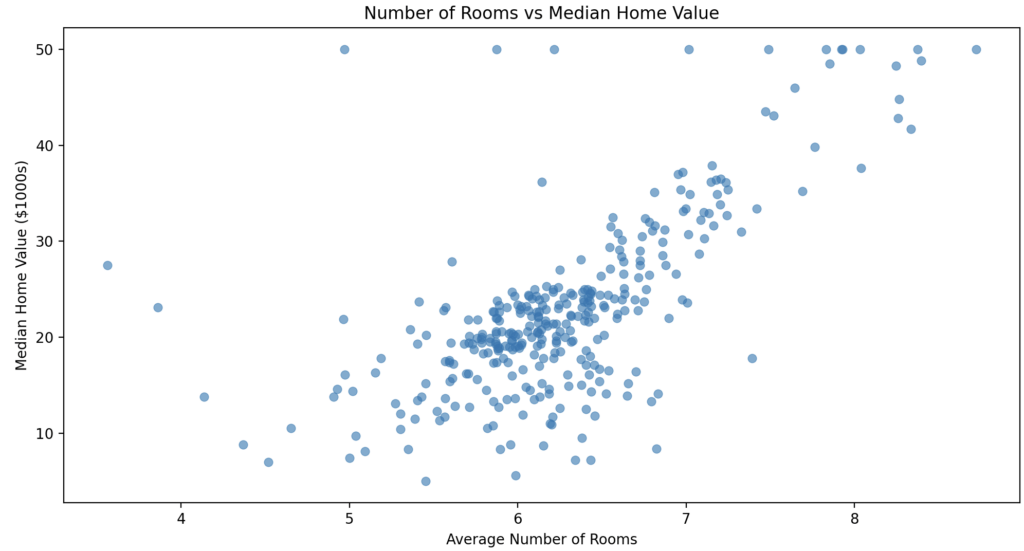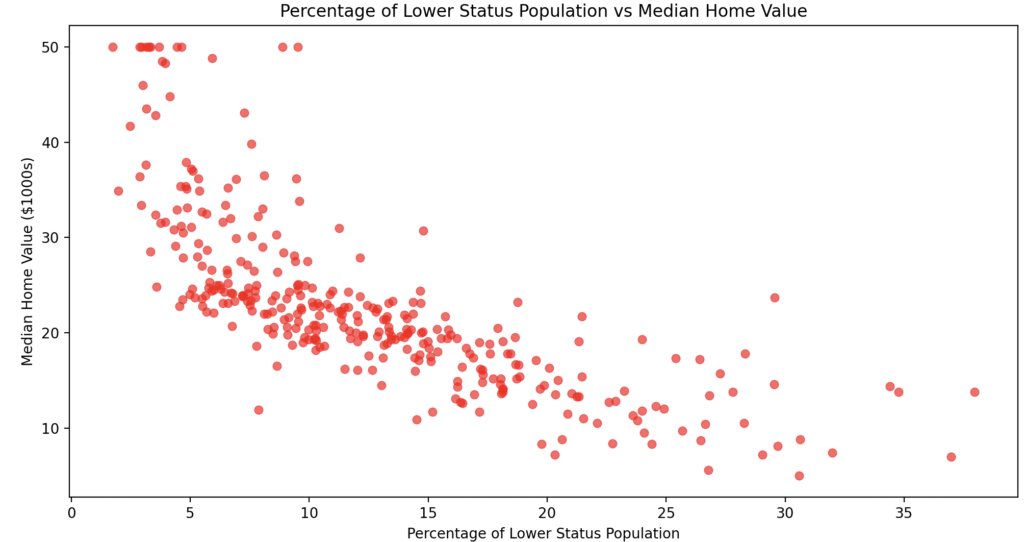### Step 7: Model Building and Training

Now that we understand our data well, let’s build our linear regression model.

For simplicity, we’ll first build a univariate linear regression model using just one feature (rm) to predict the target (medv). Later on, we can expand to include more features.

Here are the steps you need to perform.

#### 7.1: Split the data into training and test sets.

``````import pandas as pd

from sklearn.model_selection import train_test_split
from sklearn.linear_model import LinearRegression
from sklearn.metrics import mean_squared_error, r2_score

# Features and target variable
X = data[['rm']] # Using only 'rm' feature for now
y = data['medv']

# Splitting the data into training and test sets (70% train, 30% test)
X_train, X_test, y_train, y_test = train_test_split(
X, y, test_size=0.3, random_state=42)``````

#### 7.2: Train a linear regression model using the training set.

``````# Training the linear regression model
lr = LinearRegression()
lr.fit(X_train, y_train)
``````

#### 7.3: Predicting the target for the test set

``````# Predicting the target for the test set
y_pred = lr.predict(X_test)
``````

#### 7.4: Model evaluation

``````# Model evaluation
mse = mean_squared_error(y_test, y_pred)
r2 = r2_score(y_test, y_pred)

print(mse, r2)
``````

Output

``````39.15291965406773 0.4891664703846452
``````

The model’s performance metrics are as follows:

#### Mean Squared Error (MSE): 39.15

MSE measures the average squared difference between the predicted and actual values.

Lower values indicate a better fit.

#### R-squared(R2): 0.4891

R2 measures the proportion of the variance in the dependent variable that is predictable from the independent variable(s).

It ranges from 0 to 1, with higher values indicating a better fit.

R2 of 0.489 means that our model explains approximately 48.9% of the variability in the median home values.

### Step 8: Visualize the regression line on the test data

``````# Plot the regression line on the test data
plt.figure(figsize=(12, 6))
sns.scatterplot(x=X_test['rm'], y=y_test, color='blue', label='Actual Values', alpha=0.6)
plt.plot(X_test['rm'], y_pred, color='red', label='Regression Line')
plt.title('Regression Line on Test Data')
plt.xlabel('Average Number of Rooms')
plt.ylabel('Median Home Value (\$1000s)')
plt.legend()
plt.show()``````

Output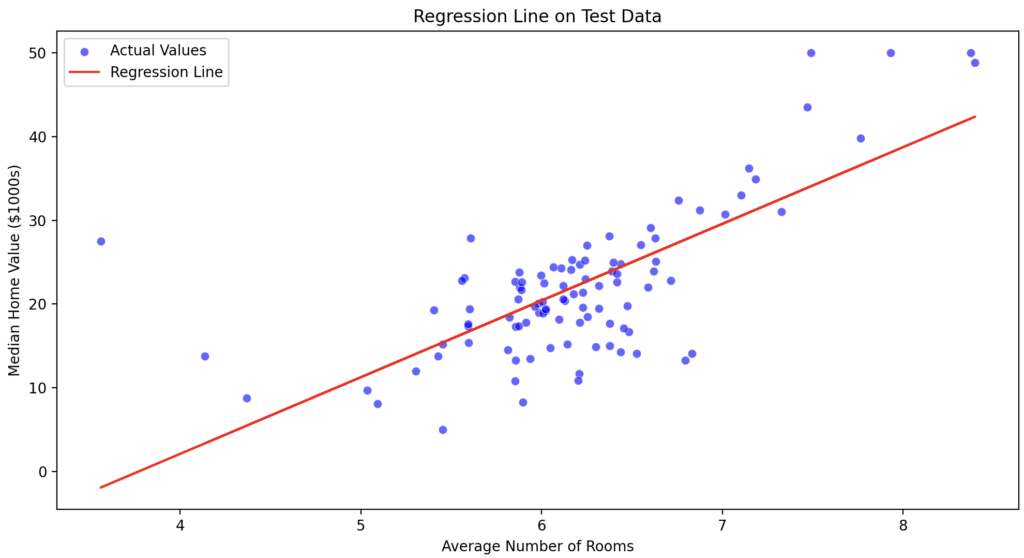The red line in the plot represents the regression line predicted by our linear regression model, while the blue dots represent the actual data points in the test set.

As we can see, the regression line does a reasonably good job of capturing the general trend in the data, but there’s room for improvement.

### Step 9: Model Evaluation

We have already computed our model’s Mean Squared Error (MSE) and R2 score. While our model explains about 48.9% of the variability in median home values (based on the R2 score), this relatively simple model uses only one feature.

We can improve the model’s performance by incorporating more features and possibly using more advanced regression techniques.

## Conclusion

In this project guide, we walked through the process of building a Simple Linear Regression Model using the Boston Housing dataset.

We started with setting up the environment, followed by data collection, pre-processing, exploratory data analysis, model building, and evaluation.

You can find this project’s code on GitHub.

This site uses Akismet to reduce spam. Learn how your comment data is processed.